## ggrastr

### Convert any ggplot2 layer into a rasterized output

Using the function `rasterize()`, users can rasterize any ggplot2 layer:

``````library(ggplot2)
library(ggrastr)

plot <- ggplot(diamonds, aes(carat, price, colour = cut))

plot + rasterise(geom_point(), dpi = 72) + theme(aspect.ratio = 1)
``````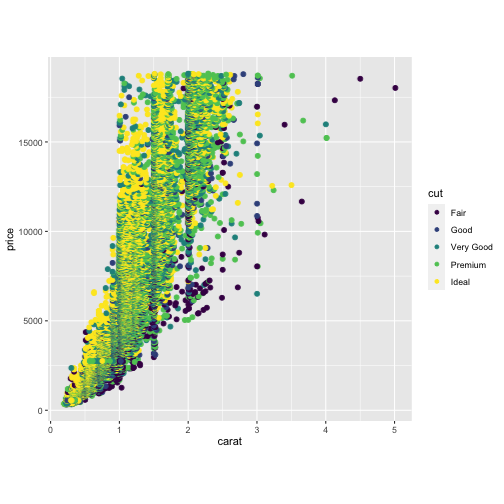Note that with ggrastr changes in version 0.2.0, when the aspect ratio is distorted, points are still rendered without distortion, i.e. the points are still circles:

``````# Points remain round across different aspect ratios
plot + rasterise(geom_point(), dpi = 72) + theme(aspect.ratio = 0.2)
``````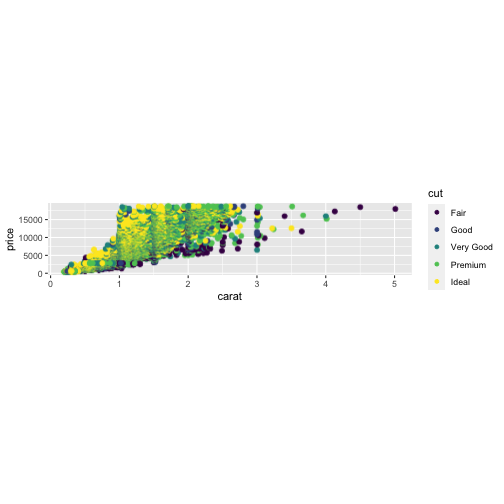By default, plots are rendered with cairo. However, users now have the option to render plots with the ragg device. The motivation for using `ragg` is that `ragg` can be faster and has better anti-aliasing. That being said, the default ragg device also has some alpha blending quirks. Because of these quirks, users are recommended to use the `ragg_png` option to work around the alpha blending.

The differences in devices are best seen at lower resolution:

``````# The default 'cairo' at dpi=5
plot + rasterise(geom_point(), dpi = 5, dev = "cairo")
``````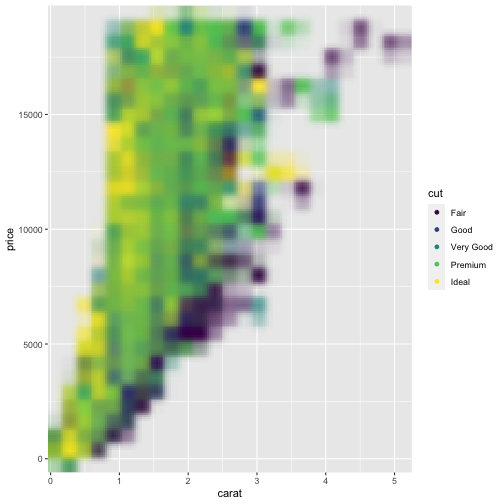``````# Using 'ragg' gives better anti-aliasing but has unexpected alpha blending
plot + rasterise(geom_point(), dpi = 5, dev = "ragg")
``````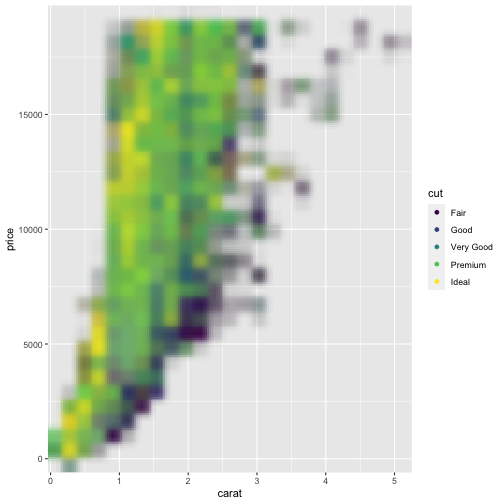``````# Using 'ragg_png' solves the alpha blend, but requires writing a temporary file to disk
plot + rasterise(geom_point(), dpi = 5, dev = "ragg_png")
``````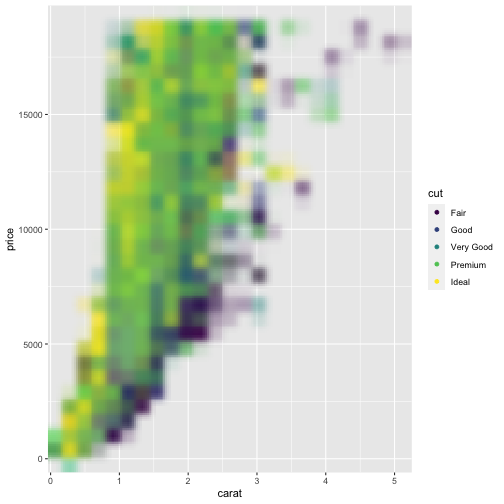### Raserized plots with facet_wrap()

Facets are rendered correctly without users having to adjust the width/height settings.

``````# Facets won't warp points
set.seed(123)
plot + rasterise(geom_point(), dpi = 300) + facet_wrap(~ sample(1:3, nrow(diamonds), 2))
``````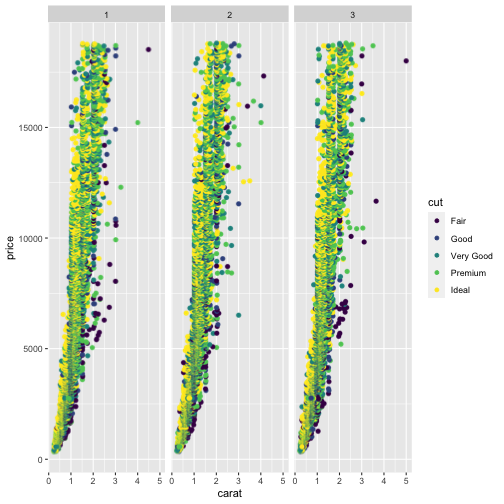### Points: Rasterize scatterplots with geom_point_rast()

Sometimes you need to publish a figure in a vector format:

``````library(ggplot2)
library(ggrastr)

points_num <- 10000
df <- data.frame(x=rnorm(points_num), y=rnorm(points_num), c=as.factor(1:points_num %% 2))
gg <- ggplot(df, aes(x=x, y=y, color=c)) + scale_color_discrete(guide=FALSE)

gg_vec <- gg + geom_point(size=0.5)
print(gg_vec)
``````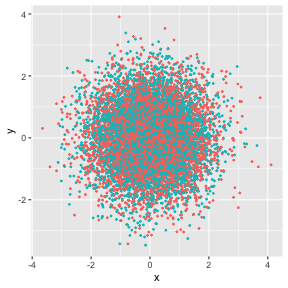But in other cases, your figure contains thousands of points, e.g. try `points_num <- 500000` in the example above, and you will notice the performance issues—it takes significantly longer to render the plot: